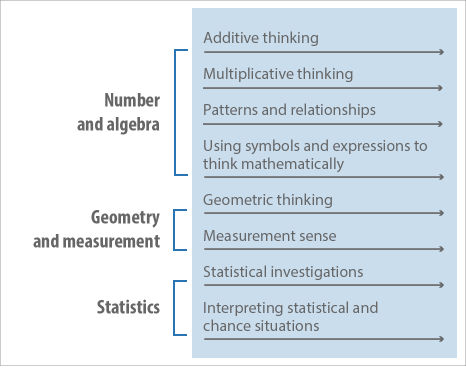# The Learning Progression Frameworks (LPFs):Understanding Progress

Understanding the mathematics framework

# The Learning Progression Frameworks (LPFs): Understanding Progress

Understanding the mathematics framework

## Why would I use the mathematics framework?

The mathematics framework supports you to develop your understanding of the big ideas of mathematics and statistics that students need to learn.
Research in mathematics education has consistently shown that a deep knowledge of mathematics and statistics and an understanding of how students learn mathematics and statistics are essential for being an effective teacher. The mathematics framework develops your content and pedagogical knowledge by providing a clear view of how students' learning develops over time in eight aspects (big ideas) of mathematics and statistics.

The progressions in the mathematics framework set out the significant steps that students take as they develop their expertise in all aspects of mathematics and statistics.
All students will need to move past the same signposts as they develop their expertise in mathematics and statistics, although they might take different amounts of time and follow slightly different pathways in between these signposts.

The mathematics framework provides a big-picture map to help you understand what progress in mathematics and statistics looks like. It illustrates this progress by showing how students respond when problems become more complex and they need to apply their skills and knowledge in more complex ways.The mathematics framework supports the implementation of the mathematics and statistics learning area by showing how important mathematical and statistical aspects (big ideas) develop.

The eight aspects of the mathematics framework cover the breadth of the mathematics and statistics learning area and emphasise making sense of mathematical ideas and reasoning mathematically. Four of the aspects address key ideas in the number and algebra strand, two address the geometry and measurement strand, and two address the statistics strand.

Student expertise is clearly communicated in annotated work samples, called illustrations. The illustrations clearly show the level of expertise expected of students at each signpost and provide examples of rich learning tasks.

## How would I use the mathematics framework?

The mathematics framework helps you to plan your classroom programme. It prompts you to consider the extent to which your programme is providing opportunities for learning across all aspects of mathematics and statistics.
It is important that students develop their knowledge and skills across all aspects of the mathematics framework so that they can use mathematics and statistics confidently, within the mathematics and statistics learning area, in other learning areas, and in their everyday lives.

The strong connections that exist among the mathematics aspects mean that lack of exposure to all the big ideas in mathematics and statistics can restrict students’ progress. The framework helps you to think about the breadth of your mathematics and statistics programme and provides a way to check that you are planning for a comprehensive coverage of the learning area.

The mathematics framework prompts you to consider what to notice as you think about how well a student is progressing in mathematics and statistics.
The illustrations help you identify your students’ current level of expertise by showing you what to pay attention to as they apply their knowledge and skills to solve problems and model situations. The signpost descriptions show what progress looks like and where your students are heading. You can use this understanding to plan the learning programme that will best help them to get to the next signpost.

The illustrations highlight tasks that focus on conceptual understanding and promote reasoning and problem solving.
The illustrations exemplify rich classroom tasks familiar to many teachers. The illustrations emphasise that students’ learning is most effective when they engage in tasks that require them to think, reason, make sense, and explain or justify their responses. The illustrations also show how, by allowing multiple entry points and varied strategies for solving problems, rich learning tasks provide challenges for students who have a range of knowledge and skills.

Understanding the mathematics framework.
Michelle from Kairanga School describes the impact of the mathematics framework on teachers' knowledge.
Understanding the mathematics framework
Kris from Otago Girls' High School describes how the mathematics framework helped teachers in their department clarify expectations for student progress.
Jeanette from Otago Girls' High School describes how the framework supports teachers to focus on what learning is happening in the classroom.
Kris from Otago Girls' High School talks about the support that the frameworks can offer beginning teachers, and those that are not as familiar with the NZC.
How to navigate the mathematics framework.
The components of a mathematics illustration.